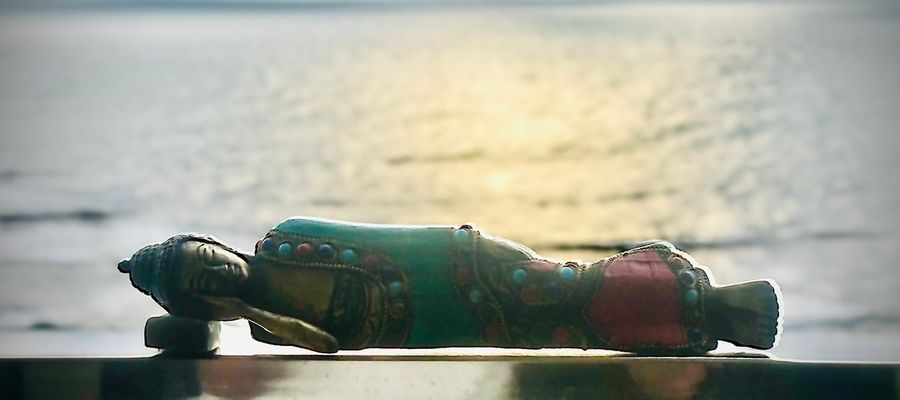# HOW WELL DO YOU KNOW YOUR IKIGAI QUOTIENT ZONE by HQGPL® 2020

## This self introspection questionnaire will guide you to reflect on the ten key elements of Ikigai as one purposeful way to find your current Ikigai quotient zone in the life journey. If you don’t know what your Ikigai is yet, your mission is to discover it.### Quiz Questions

• 1.
HOW ACTIVE IS YOUR LIFESTYLE ? Rate yourself on a 1 - 10 scale where 1 is the lowest and 10 is the highest. The total points would reveal the Ikigai Zone..
• A.
1
• B.
2
• C.
3
• D.
4
• E.
5
• F.
6
• G.
7
• H.
8
• I.
9
• J.
10
• 2.
HOW PATIENT ARE YOU? 1 being very impatient and 10 being very patient. Rate yourself on a 1 - 10 scale where 1 is the lowest and 10 is the highest. The total points would reveal the Ikigai Zone.
• A.
1
• B.
2
• C.
3
• D.
4
• E.
5
• F.
6
• G.
7
• H.
8
• I.
9
• J.
10
• 3.
DO YOU KNOW YOUR PURPOSE YET? 1 being no idea and 10 being very good idea. Rate yourself on a 1 - 10 scale where 1 is the lowest and 10 is the highest. The total points would reveal the Ikigai Zone..
• A.
1
• B.
2
• C.
3
• D.
4
• E.
5
• F.
6
• G.
7
• H.
8
• I.
9
• J.
10
• 4.
HOW OFTEN DO YOU SMILE IN A DAY? 1 being less than 5 times a day and 10 being more than 50 times a day. Rate yourself on a 1 - 10 scale where 1 is the lowest and 10 is the highest. The total points would reveal the Ikigai Zone
• A.
1
• B.
2
• C.
3
• D.
4
• E.
5
• F.
6
• G.
7
• H.
8
• I.
9
• J.
10
• 5.
HOW OFTEN ARE YOU CONNECTING WITH NATURE*? 1 being less than once a year and 10 being more than 10 times a year. Rate yourself on a 1 - 10 scale where 1 is the lowest and 10 is the highest. The total points would reveal the Ikigai Zone.. * Trek, Camping, Outdoor Adventure, Wild life Sanctuary, Long Distance Cycling,etc
• A.
1
• B.
2
• C.
3
• D.
4
• E.
5
• F.
6
• G.
7
• H.
8
• I.
9
• J.
10
• 6.
HOW MANY TIMES A DAY DO YOU GIVE THANKS? LESS THAN 5 per day being 1 AND 10 being MORE THAN 50 IN A DAY. Rate yourself on a 1 - 10 scale where 1 is the lowest and 10 is the highest. The total points would reveal the Ikigai Zone.
• A.
1
• B.
2
• C.
3
• D.
4
• E.
5
• F.
6
• G.
7
• H.
8
• I.
9
• J.
10
• 7.
DESCRIBE HOW MUCH % OF THE DAY DO YOU SPEND LIVING IN THE MOMENT? LESS THAN 10% being 1 AND 10 being MORE THAN 90%. Rate youself on a 1 - 10 scale where 1 is the lowest and 10 is the highest. The total points would reveal the Ikigai Zone..
• A.
1
• B.
2
• C.
3
• D.
4
• E.
5
• F.
6
• G.
7
• H.
8
• I.
9
• J.
10
• 8.
HOW DO YOU RATE YOUR FOOD HABITS IN TERMS OF QUALITY AND QUANTITY? POOR QUALITY AND HIGH QUANTITY being 1 AND 10 being HIGH QUALITY AND RIGHT QUANTITY. Rate the yourself on a 1 - 10 scale where 1 is the lowest and 10 is the highest. The total points would reveal the Ikigai Zone.
• A.
1
• B.
2
• C.
3
• D.
4
• E.
5
• F.
6
• G.
7
• H.
8
• I.
9
• J.
10
• 9.
RATE YOUR FITNESS LEVELS IN RELATION TO THEIR AGE AND TARGET GOALS. VERY UNFIT AND FAR FROM THE GOAL being 1 AND 10 being VERY FIT AND IN LINE WITH THE GOAL. Rate yourself on a 1 - 10 scale where 1 is the lowest and 10 is the highest. The total points would reveal the Ikigai Zone...
• A.
1
• B.
2
• C.
3
• D.
4
• E.
5
• F.
6
• G.
7
• H.
8
• I.
9
• J.
10
• 10.
RATE YOUR CIRCLE OF FRIENDS IN TERMS OF QUALITY. UNSATISFACTORY being 1 AND 10 being MEANINGFUL. Rate this on a 1 - 10 scale where 1 is the lowest and 10 is the highest. The total points would reveal the Ikigai Zone.
• A.
1
• B.
2
• C.
3
• D.
4
• E.
5
• F.
6
• G.
7
• H.
8
• I.
9
• J.
10

• 1.
RED ZONE